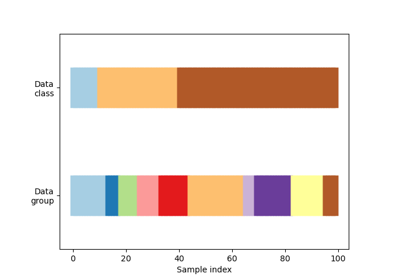# sklearn.model_selection.GroupShuffleSplit¶

class sklearn.model_selection.GroupShuffleSplit(n_splits=5, test_size=None, train_size=None, random_state=None)[source]

Shuffle-Group(s)-Out cross-validation iterator

Provides randomized train/test indices to split data according to a third-party provided group. This group information can be used to encode arbitrary domain specific stratifications of the samples as integers.

For instance the groups could be the year of collection of the samples and thus allow for cross-validation against time-based splits.

The difference between LeavePGroupsOut and GroupShuffleSplit is that the former generates splits using all subsets of size p unique groups, whereas GroupShuffleSplit generates a user-determined number of random test splits, each with a user-determined fraction of unique groups.

For example, a less computationally intensive alternative to LeavePGroupsOut(p=10) would be GroupShuffleSplit(test_size=10, n_splits=100).

Note: The parameters test_size and train_size refer to groups, and not to samples, as in ShuffleSplit.

Parameters: n_splits : int (default 5) Number of re-shuffling & splitting iterations. test_size : float, int, None, optional (default=None) If float, should be between 0.0 and 1.0 and represent the proportion of the dataset to include in the test split. If int, represents the absolute number of test groups. If None, the value is set to the complement of the train size. If train_size is also None, it will be set to 0.2. train_size : float, int, or None, default is None If float, should be between 0.0 and 1.0 and represent the proportion of the groups to include in the train split. If int, represents the absolute number of train groups. If None, the value is automatically set to the complement of the test size. random_state : int, RandomState instance or None, optional (default=None) If int, random_state is the seed used by the random number generator; If RandomState instance, random_state is the random number generator; If None, the random number generator is the RandomState instance used by np.random.

Methods

 get_n_splits(self[, X, y, groups]) Returns the number of splitting iterations in the cross-validator split(self, X[, y, groups]) Generate indices to split data into training and test set.
__init__(self, n_splits=5, test_size=None, train_size=None, random_state=None)[source]
get_n_splits(self, X=None, y=None, groups=None)[source]

Returns the number of splitting iterations in the cross-validator

Parameters: X : object Always ignored, exists for compatibility. y : object Always ignored, exists for compatibility. groups : object Always ignored, exists for compatibility. n_splits : int Returns the number of splitting iterations in the cross-validator.
split(self, X, y=None, groups=None)[source]

Generate indices to split data into training and test set.

Parameters: X : array-like, shape (n_samples, n_features) Training data, where n_samples is the number of samples and n_features is the number of features. y : array-like, shape (n_samples,), optional The target variable for supervised learning problems. groups : array-like, with shape (n_samples,) Group labels for the samples used while splitting the dataset into train/test set. train : ndarray The training set indices for that split. test : ndarray The testing set indices for that split.

Notes

Randomized CV splitters may return different results for each call of split. You can make the results identical by setting random_state to an integer.

## Examples using sklearn.model_selection.GroupShuffleSplit¶Visualizing cross-validation behavior in scikit-learn